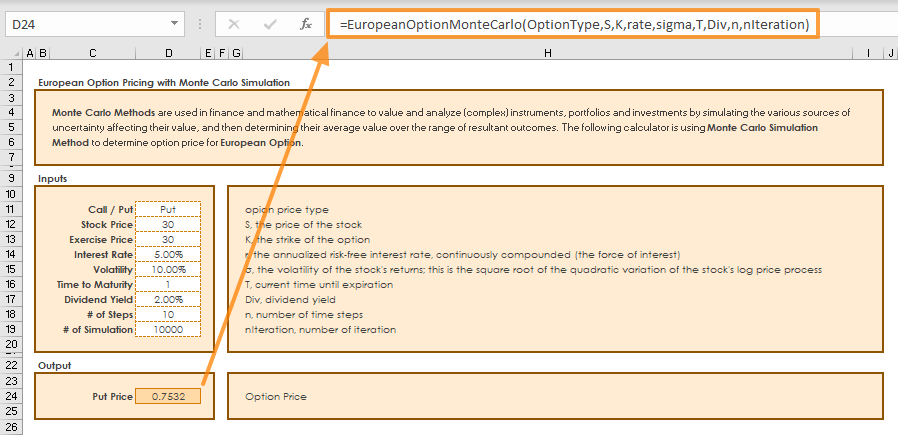In finance, option pricing is a term used for estimating the value of an option contract using all known inputs. Monte Carlo Simulation is a popular algorithm that can generate a series of random variables with similar properties to simulate realistic inputs. In this guide, we're going to show you how to calculate Option Pricing using Monte Carlo Simulations in Excel.

## Monte Carlo Simulation

Monte Carlo simulation is a special type of probability simulation which is mainly used to determine the risk factors by observing the cluster of possible results. First developed for finding the possible outcomes of a solitaire game, Monte Carlo takes its name from the famous casino in Monaco.

The simulation takes random values of the inputs within constraints and the results are recorded as more iterations are run. Then, you get a rather big pool of answers created from all those random inputs.

We can simulate the possible future stock prices and then use them to find the discounted expected option payoffs.

Using the statistical formulas NORM.S.INV and VBA, we can generate random variables in normal distribution and run the simulation as many times as necessary..

## European-style Options Pricing

In this example, we are going to be using the Black-Scholes formula to calculate a European-style option pricing model, which restricts its options execution until the expiration date. There are two major types of options: calls and puts.

• Call is an option contract between the buyer and the seller of the call option, to exchange a security at a set price.
• Put is an option contract which gives the purchaser of the put option the right to sell an asset, at a specified price, by a specified date to the seller of the put.

The Black-Scholes formula is a popular approach for calculating European put and call options. In its simplest form, the Black-Scholes model involves underlying assets of a risk-free rate of return and a risky share price. The following equation shows how a stock price varies over time:St = Stock price at time t

r = Risk-free rate

t = time

σ = T he volatility of the stock's returns; this is the square root of the quadratic variation of the stock's log price process

ε = random generated variable from a normal distribution

δ = Dividend yield which was not in the Black-Scholes model originally. The original model was for pricing options on non-paying dividends stocks.

Once the formula is run thousands or million times, you will have the set of St values. The payoff values can be calculated with the following formula, where K is the strike price:## Calculating Option Pricing with VBA

Let’s pass these formulations into a VBA code. We are going to create a user defined function (UDF) which can be used as a built-in function like SUM or VLOOKUP. Our function name is “EuropeanOptionMonteCarlo”.

```Public Function EuropeanOptionMonteCarlo(OptionType As String, S As Double, K As Double, r As Double, sigma As Double, T As Double, Div As Double, N As Double, nIt As Double)

Dim i As Integer, j As Integer
Dim Payoff() As Double, St As Double, dt As Double, e As Double, price As Double

ReDim Payoff(1 To nIt)

dt = T / N

Randomize 'new seed for random number generation

For i = 1 To nIt
St = S
For j = 1 To N
e = WorksheetFunction.NormSInv(Rnd()) 'random factor
St = St * Exp((r - Div - sigma ^ 2 / 2) * dt + sigma * Sqr(dt) * e) 'European option formula
Next j

If OptionType = "Call" Then 'Call or Put
Payoff(i) = WorksheetFunction.Max(St - K, 0) * Exp(-r * T)
ElseIf OptionType = "Put" Then
Payoff(i) = WorksheetFunction.Max(K - St, 0) * Exp(-r * T)
End If

Next i

For i = 1 To nIt
price = price + Payoff(i) 'Total of iterations
Next i

EuropeanOptionMonteCarlo = price / nIt 'Return average of iterations as the function's result

End Function```

Once the UDF is ready, we are ready to see the result in Excel.